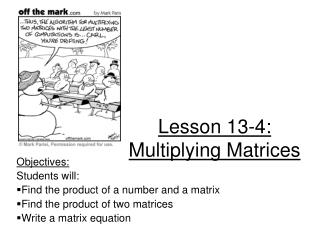DownloadDownload PresentationLesson 13-4: Multiplying Matrices

# Lesson 13-4: Multiplying Matrices

Télécharger la présentation## Lesson 13-4: Multiplying Matrices

- - - - - - - - - - - - - - - - - - - - - - - - - - - E N D - - - - - - - - - - - - - - - - - - - - - - - - - - -
##### Presentation Transcript

1. Lesson 13-4: Multiplying Matrices Objectives: Students will: Find the product of a number and a matrix Find the product of two matrices Write a matrix equation

2. Multiplying by Scalar: Denoted kA Distribute “k” to every number in matrix Matrices: ● Add products of 1st matrix’s rows & 2nd matrix’s column ● Write results in columns

3. Rules: When multiplying: m x n ● n x p Columns in 2nd matrix = columns in answer Must match m x p → Answer matrix

4. Example 1:

5. Example 2 The solution will be 3 x 1 3 X 3 3X1

6. Try It 3

7. Ex 4

8. Writing Matrix Equations 5x + 3y + 2z = 7 4x + 8y + 9z = 1 2x + y + 7z = 3 Coefficient Matrix Variable Matrix Constant matrix ● = Coefficients in first matrix → I see if I multiply the matrices, I will get the equations I started with!! Variable in second matrix Constants here!